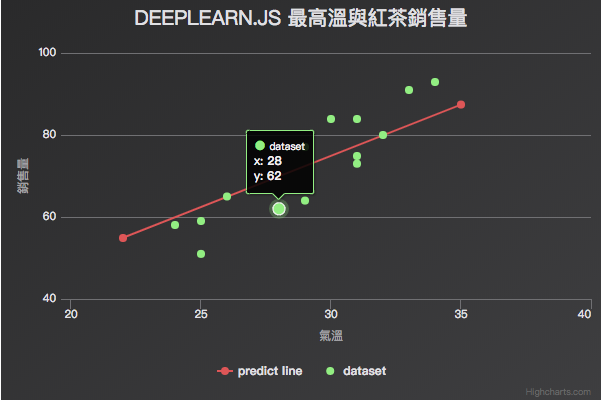# 前言## 先進入假想情境！

Y = aX + b

## 先別急，讓我們先把資料整理一下

// 建構資料 （當然是假的）
const degrees = [29, 28, 34, 31, 25, 29, 32, 31, 24, 33, 25, 31, 26, 30];
const salesVolume = [77, 62, 93, 84, 59, 64, 80, 75, 58, 91, 51, 73, 65, 84];


See the Pen DeeplearnJS-simple-linear-dataset by Arvin (@arvin0731) on CodePen.

## 解法都有了，那就來看看到底怎麼用 Javascript 實作吧！

<script src="https://unpkg.com/deeplearn@latest"></script>


// 建構資料
const degrees = [29, 28, 34, 31, 25, 29, 32, 31, 24, 33, 25, 31, 26, 30];
const salesVolume = [77, 62, 93, 84, 59, 64, 80, 75, 58, 91, 51, 73, 65, 84];
// 運用 Deeplearn.js 結構化資料
// dl 就是 deeplearn.js 的 global 變數
const degrees_data = dl.tensor1d(degrees);
const salesVolume_data = dl.tensor1d(salesVolume);


• values (TypedArray|Array): tensor 的值。可以是 nested array 或 flat array 的結構。

• shape（number[]）:基本上就是該 tensor 的維度。若創建 tensor 時沒有指定維度，就會繼承傳入的 values 維度。也可以像這邊的範例一樣直接使用 tensor\${1|2|3|4}d 來創建

• dtype（float32'|'int32'|'bool）：值的型別，當然是 optional。

// 要 train 的參數 aw, ba
const aw = dl.variable(dl.scalar(Math.random()));
const ba = dl.variable(dl.scalar(Math.random()));


dl.variable(initialValue, trainable?, name?, dtype?) 用來創建 training 過程中需要的變數，也可透過參數指定該變數能否在 training 過程中被修改（trainable），預設是 true

dl.scalar 是維度為 0 的 tensor，基本上 0 維就是一個點，由於我們只需要亂數產生一個初值給 awba，因此用 dl.scalar 即可。

// 定義目標函數 與 loss function （最一般的 mean square）
// f = aw * X + ba
const f = x => aw.mul(x).add(ba);
const loss = (pred, label) => pred.sub(label).square().mean();


loss function 則是接收兩個參數的函式，pred 就是 training data 中的自變因 x 透過目標函數 f，計算出的值，而 label 是 training data 中的答案 y

// 採用 stochastic gradient descent 來做最佳化
// learning rate 這邊不能設太大
const learningRate = 0.0005;
const optimizer = dl.train.sgd(learningRate);


dl.train.sgd 是 deeplearn.js 內建的 sgd 演算法模型，接受一個 leanring rate 參數。在每一次的迭代中，係數都會不斷被更新，以找出最佳的結果，而這個 learningRate 參數是用來控制每一次的更新幅度。因此不能夠設得太大，也不能設得太小。

// training
for (let i = 0; i < 30; i++) {
const cost = optimizer.minimize(() => loss(f(degrees_data), salesVolume_data), true, [aw, ba])
console.log('cost');
cost.print();
console.log('aw');
aw.print();
console.log('ba');
ba.print();
}


const cost = optimizer.minimize(() => loss(f(degrees_data), salesVolume_data), true, [aw, ba])

// 利用 dataSync() 取出 training 後得到的係數
const awPredict = aw.dataSync();
const baPredict = ba.dataSync();


const dataLine = [
[22, parseFloat(22 * awPredict + baPredict)],
[35, parseFloat(35 * awPredict + baPredict)]
]


const options = {
title: {
text: 'deeplearn.js  最高溫與紅茶銷售量'
},
xAxis: {
title: {
text: '氣溫'
},
min: 20,
max: 40
},
yAxis: {
title: {
text: '銷售量'
},
min: 40,
max: 100
},
series: [
{
type: 'line',
name: 'predict line',
data: dataLine
},
{
type: 'scatter',
name: 'dataset',
marker: {
symbol: 'cross',
},
data: dataset
}
]
}
// 繪製圖表
const chart = Highcharts.chart('app', options);


## 最終成果

See the Pen DeeplearnJS-simple-linear by Arvin (@arvin0731) on CodePen.

## 資料來源

@arvinh 前端攻城獅，熱愛數據分析和資訊視覺化